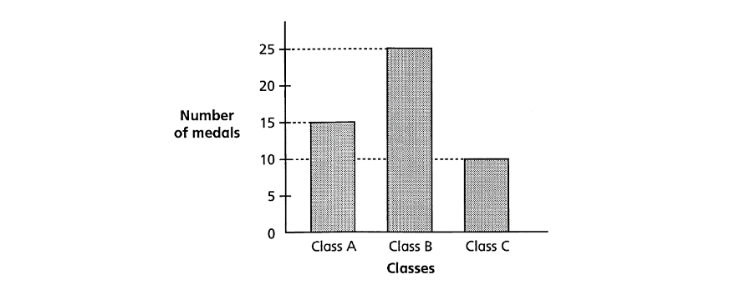top of page
Search

# 5 Tips to Master Primary 3 Math’ Bar GraphsPrimary 3 Maths introduces exciting concepts, and one of the challenging Primary 3 Maths topics is bar graphs. Bar graphs are essential for visualising data and understanding patterns. In this article, we'll explore five tips to help Primary 3 students grasp the concept of bar graphs and excel in interpreting and creating them.

## Understanding the Basics: What is a Bar Graph in Math?

Before delving into the tips, let's establish a solid understanding of what a bar graph is. In Mathematics, it's a visual representation of data using bars of varying heights or lengths. These bars represent different categories, and their sizes correspond to the values of those categories. Bar graphs are widely used to compare different data sets or to show data changes over time. They can be drawn vertically or horizontally.### Tip 1: Grasp the Concept of Bar Graphs

Mastering bar graphs begins with understanding their concept. Think of these graphs as visual stories conveying information. Each bar represents a specific category, and its length or height corresponds to the data quantity. To interpret them accurately, focus on the relationship between categories and their values.### Tip 2: Understand the Components of a Bar Graph

A bar graph comprises two main axes – a vertical axis and a horizontal axis. In the example below, the vertical axis indicates the scale, i.e., the units or intervals the graph is divided into. The horizontal axis represents the compared categories. In simple terms, the vertical axis gives "how much" information, while the horizontal axis provides the "what" information.### Tip 3: Label the Bars

Precision is crucial when working with bar graphs. Each bar must be accurately labelled to avoid confusion. This involves placing the correct value above each bar, ensuring it's centred and legible. Proper labelling aids in tracking your thought process and helps your teacher understand how you arrived at your answers. The labels above the bars serve as a clear and concise way to communicate your findings.### Tip 4: Carefully Read the Question

Before solving a bar graph-related problem, carefully read and understand the question. Look for keywords guiding you to identify the required information. For instance, if the question seeks a total, calculate that total accurately based on the data provided. Decode the question's intent to extract necessary data from the graph.Solutions:

5 × 0 = 0

9 × 1 = 9

18 × 2 = 36

12 × 3 = 36

20 × 4 = 80

9 + 36 + 36 + 80 = 161

You cannot just add all the numbers together. That will give you the total number of students.

5 students borrowed 0 books,

9 students borrowed 1 book,

18 students borrowed 2 books,

12 students borrowed 3 books and

20 students borrowed 4 books.

You need to multiply them respectively in order to find the total number of books borrowed by all the students.

### Tip 5: Create Your Own Bar Graphs

To truly master working with bar graphs, practice is essential. An effective way is to create your own bar graphs using gathered or provided data. This hands-on approach reinforces your graphing skills and provides a clearer understanding of constructing a bar graph.

Example: Create a bar graph to show the number of medals earned by each class using that data as follows:

• Class A: 15 medals

• Class B: 25 medals

• Class C: 10 medals

To create a bar graph:

1. Draw a horizontal line and a vertical line.

2. Label the horizontal line with the names of the students and the vertical line with the number of medals.

3. Mark off appropriate intervals on the vertical line (for example, 5, 10, 15, 20, 25).

4. Draw a rectangular bar above each student's name, with the height of the bar corresponding to the number of medals earned.

Here's what the finished bar graph might look like:## Real-Life Application of Bar Graphs

Understanding practical applications is essential while learning bar graphs. They hold real-life significance beyond Mathematics. Think of scenarios like tracking students' book reading, comparing product sales, or displaying city populations. In such cases, bar graphs become powerful tools for summarising and presenting data visually.

In conclusion, mastering bar graphs in Primary 3 Maths involves understanding their concept, comprehending axes and labels, careful reading of Primary 3 Maths questions, and hands-on practice. Bar graphs aren't just exercises; they're connected to the world around us. As Primary 3 students embrace these tips, they enhance Mathematical skills and develop valuable analytical perspectives extending beyond the classroom. So, start graphing and witness the data world unfold!Seize the opportunity and enrol your child at AGrader Learning Centre! Parents of Primary 3 students, if you aim to give your child an edge in mastering Maths, search no more.

AGrader Learning Centre offers exceptional Primary Maths tuition, tailored for Primary 1 to 6 students. With unwavering commitment to excellence, our Primary School Maths tuition aligns seamlessly with the latest Ministry of Education syllabus.

Act now to elevate your child's Maths skills! Enrol in AGrader Learning Centre's Primary Math Tuition programme, equipping your child for a bright academic future.